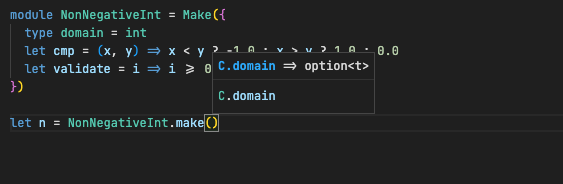# Module type and intellisense looks weird after functor

I’m using functors to generate validated primitive types, like a `NonNegativeInt`. It works. But the tooltip/intellisense for using the functor is kind of strange. For example, in the code below when I try to use my `NonNegativeInt` method here is what I see…What I want to see is `int` instead of `C.domain`. At this point, what is module C? If I want all traces of `C.domain` to be replaced with `int` I think I need to do the `BetterNonNegativeInt` casting/assignment below, which is an extra step. Is there some way to do all this in a single step? Is this a problem with the VS Code extension?

``````module type T = {
type t
type domain
let eq: (t, t) => bool
let make: domain => option<t>
let value: t => domain
}

module type Configuration = {
type domain
let cmp: (domain, domain) => float
let validate: domain => option<domain>
}

module Make = (C: Configuration): (T with type domain := C.domain) => {
type t = C.domain
type domain = C.domain
let eq = (i: t, j: t) => C.cmp(i, j) === 0.0
let value = i => i
let make = (i: domain) => C.validate(i)
}

module NonNegativeInt = Make({
type domain = int
let cmp = (x, y) => x < y ? -1.0 : x > y ? 1.0 : 0.0
let validate = i => i >= 0 ? Some(i) : None
})

module BetterNonNegativeInt: T with type domain := int = NonNegativeInt
``````

These kinds of issues are better to post on the issue tracker for the VSCode extension rather than on the forums.

Ok no problem. I just wasn’t sure if there was something wrong or whether I’m not understanding how functors work.

I made a post on that github issue you opened adding some screenshots for context.

But if you want a quick fix (and someone reading this thread is interested), you can write your `NonNegativeInt` module like this:

``````module NonNegativeInt: T with type domain := int = Make({
type domain = int
let cmp = (x, y) => x < y ? -1.0 : x > y ? 1.0 : 0.0
let validate = i => i >= 0 ? Some(i) : None
})
``````

with the explicit signature there and you will get the correct type hits and signatures.